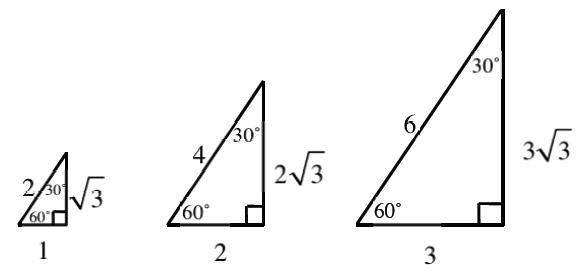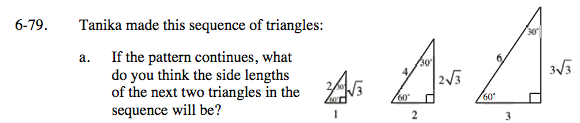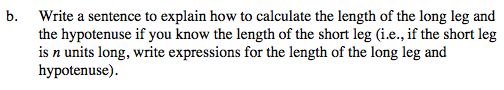### Home > INT3 > Chapter 6 > Lesson 6.3.1 > Problem6-79

6-79.
1.Tanika made this sequence of triangles: Homework Help ✎

1. If the pattern continues, what do you think the side lengths of the next two triangles in the sequence will be?

2. Write a sentence to explain how to calculate the length of the long leg and the hypotenuse if you know the length of the short leg (i.e., if the short leg is n units long, write expressions for the length of the long leg and hypotenuse).Follow the linear patterns for each side.

$\left(4,\ 8,\ 4\sqrt{3}\right),\ \left(5,\ 10,\ 5\sqrt{3}\right)$